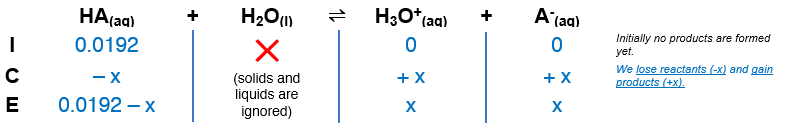# Problem: Enough of a monoprotic acid is dissolved in water to produce a 0.0192 M solution. The pH of the resulting solution is 2.53. Calculate the Ka for the acid.

###### FREE Expert Solution

The dissociation of a monoprotic weak acid (HA) is as follows:

HA(aq) + H2O(l)  H3O+(aq) + A(aq)

From this, we can construct an ICE table. Remember that liquids are ignored in the ICE table and Ka expression.90% (192 ratings)###### Problem Details

Enough of a monoprotic acid is dissolved in water to produce a 0.0192 M solution. The pH of the resulting solution is 2.53. Calculate the Ka for the acid.

Frequently Asked Questions

What scientific concept do you need to know in order to solve this problem?

Our tutors have indicated that to solve this problem you will need to apply the Weak Acids concept. If you need more Weak Acids practice, you can also practice Weak Acids practice problems.